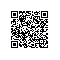# 不要再依赖 CommonJS 了

CommonJS 是 2009 年的标准，为 JavaScript 模块建立了约定。它最初打算在 Web 浏览器之外的场景中使用，主要用于服务端应用程序。

// utils.js
const { maxBy } = require('lodash-es');
const fns = {
add: (a, b) => a + b,
subtract: (a, b) => a - b,
multiply: (a, b) => a * b,
divide: (a, b) => a / b,
max: arr => maxBy(arr)
};
Object.keys(fns).forEach(fnName => module.exports[fnName] = fns[fnName]);

// index.js
const { add } = require(‘./utils');
console.log(add(1, 2));

CommonJS 如何影响最终的打包大小？

JavaScript 打包器和压缩器（minifier），例如 webpack 和 terser，会执行多种优化措施以减小应用程序的大小。它们在构建时分析你的应用程序，尝试尽可能删掉那些没用到的源代码。

const path = require('path');
module.exports = {
entry: 'index.js',
output: {
filename: 'out.js',
path: path.resolve(__dirname, 'dist'),
},
mode: 'production',
};

\$ cd dist && ls -lah
625K Apr 13 13:04 out.js

export const add = (a, b) => a + b;
export const subtract = (a, b) => a - b;
export const multiply = (a, b) => a * b;
export const divide = (a, b) => a / b;
import { maxBy } from 'lodash-es';
export const max = arr => maxBy(arr);

import { add } from './utils';
console.log(add(1, 2));

(()=>{"use strict";console.log(1+2)})();

// utils.js
export const add = (a, b) => a + b;
export const subtract = (a, b) => a - b;
// index.js
import { add } from ‘./utils';
const subtract = (a, b) => a - b;
console.log(add(1, 2));

const path = require('path');
module.exports = {
entry: 'index.js',
output: {
filename: 'out.js',
path: path.resolve(__dirname, 'dist'),
},
optimization: {
minimize: false
},
mode: 'production',
};

/******/ (() => { // webpackBootstrap
/******/     "use strict";
// CONCATENATED MODULE: ./utils.js**
const add = (a, b) => a + b;
const subtract = (a, b) => a - b;
// CONCATENATED MODULE: ./index.js**
const index_subtract = (a, b) => a - b;**
/******/ })();

• 删除未使用的 subtract 和 index_subtract 函数
• 删除所有注释和多余的空格
• 在 console.log 调用中内联 add 函数的主体
开发人员通常将这种移除未使用的导入的操作称为摇树优化（tree-shaking）。因为 webpack 能够静态地（在构建时）了解我们从 utils.js 导入及导出的符号，所以它才能实现摇树优化。

ES 模块默认启用此行为，因为与 CommonJS 相比，它们更容易进行静态分析。

// utils.js
const { maxBy } = require('lodash-es');
const fns = {
add: (a, b) => a + b,
subtract: (a, b) => a - b,
multiply: (a, b) => a * b,
divide: (a, b) => a / b,
max: arr => maxBy(arr)
};
Object.keys(fns).forEach(fnName => module.exports[fnName] = fns[fnName]);

...
(() => {
"use strict";
/* harmony import */ var _utils__WEBPACK_IMPORTED_MODULE_0__ = __webpack_require__(288);
const subtract = (a, b) => a - b;
})();

module.exports[localStorage.getItem(Math.random())] = () => { … };

【云栖号在线课堂】每天都有产品技术专家分享！

【云栖号在线课堂 社群】https://c.tb.cn/F3.Z8gvnK使用钉钉扫一扫加入圈子
+ 订阅